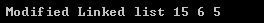# Answered! Write a code snippet to insert( or remove) a data value from a doubly-linked list….

Write a code snippet to insert( or remove) a data value from a doubly-linked list.

Here is code:

Don't use plagiarized sources. Get Your Custom Essay on
Answered! Write a code snippet to insert( or remove) a data value from a doubly-linked list….
GET AN ESSAY WRITTEN FOR YOU FROM AS LOW AS \$13/PAGE

#include <iostream>
#include <stdlib.h> /* malloc, free, rand */
using namespace std;
/* a node of the doubly linked list */
struct node
{
int data;
struct node *next;
struct node *prev;
};

void deleteNode(struct node **refNode, struct node *deleteNode)
{
// base case
if(*refNode == NULL || deleteNode == NULL)
return;

// if the node is head node
if(*refNode == deleteNode)
*refNode = deleteNode->next;

// if the node is in middle
if(deleteNode->next != NULL)
deleteNode->next->prev = deleteNode->prev;

// change the prev node
if(deleteNode->prev != NULL)
deleteNode->prev->next = deleteNode->next;
return;
}

void push(struct node** refNode, int newData)
{
// allocate node
struct node* newNode = (struct node*) malloc(sizeof(struct node));

// put in the data
newNode->data = newData;

// set the previous node to null
newNode->prev = NULL;

// set the next node to refNode
newNode->next = (*refNode);

// set the previous data to new Naode
if((*refNode) != NULL)
(*refNode)->prev = newNode ;

(*refNode) = newNode;
}

void printList(struct node *node)
{
while(node!=NULL)
{
cout << node->data << ” “;
node = node->next;
}
}

int main()
{